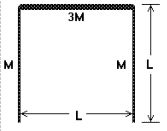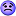# Moment of inertia with system of rods

## Homework Statement

Three slim rods with the same length L are placed in a inverted U shape, as the figure shows. The masses of the vertical rods are the same, while the third bar has the mass 3 times bigger than the first or the second rod. The rods' thickness should be ignored. Find the moment of inercia of the system in relation to the following axes:

(a) containing each bar;
(b) parallel to the plane of the page and perpendicular to the bars, going through their centers of mass;
(c) perpendicular to the plane of the page, going through the center of mass of each bar;
(d) perpendicular to the plane of the page, going through the center of mass of the system xCM = L / 2 and yCM = 4 L / 5 taking the origin as the lowest point in the left vertical bar;

Answers: (a) 2 ML2, (2/3) ML2 e 2 ML2 ; (b) (11/12) ML2 e (3/4) ML2; (c) (35/12) ML2 , (17/12) ML2 e (35/12) ML2; (d) (73/60) ML2.

(question was translated, let me know if something is difficult to understand)## Homework Equations## The Attempt at a Solution

I tried to solve question (a) considering each bar as a point, that failed however.

Calculating Icm of the first bar with an axis going through it

Icm = M(0²) + 3M(L/2)² + M(M(0²)
Icm = 3/4ML²

I tried a few variations of this but couldn't reach the answer 2 ML2, so now I'm stuck.Any help is appreciated.

#### Attachments

For some reason it gives me an errror when I try to edit the topic. Here are the correct answers:

(a) 2 ML², (2/3) ML² e 2 ML² ; (b) (11/12) ML² e (3/4) ML²; (c) (35/12) ML² , (17/12) ML² e (35/12) ML²; (d) (73/60) ML².

and M(M(0²) should be M(0²)

Nathanael
Homework Helper
Your discrete definition of rotational inertia also has a continuous form which should be used for continuous bodies, like rods.

The inertia of the middle bar about one of the edge bars comes from that integral over all the mass. The inertia is not the same if you replace the body with a point-mass at its center, which is what your math seems to be doing.

Your math also seems to say that the inertia of one edge bar about the other edge bar is zero?